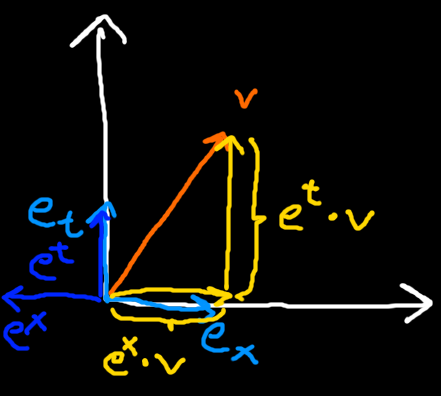### Special Relativity with Geometric Algebra - Measuring in Spacetime

In the previous section we derived the Spacetime Algebra used all over GA formualtions of physics. As a next step we need to take a look at how space and time measurements are done in it. For this we will first look at how it is done in ordinary space, and then we will try to figure out how to do it for spacetime.

#### Measuring in Ordinary Space

We want to measure the size of an object in ordinary space. The thing we want to measure has endpoints$a$and$b$, for example a stick. The endpoints can be represented by position vectors, and we can form a difference vector$v$which encodes the extents in each dimension of space.
$\begin{array}{}\text{(1)}& \begin{array}{rl}a& ={a}_{x}{e}_{x}+{a}_{y}{e}_{y}\\ b& ={b}_{x}{e}_{x}+{b}_{y}{e}_{y}\\ v& =b-a\end{array}\end{array}$
To measure the length of the stick in the X direction, we calculate the inner product with the${e}_{x}$basis vector, because then we pick out exactly the X component of the difference vector. And same for the Y component.
$\begin{array}{}\text{(2)}& \begin{array}{rl}v& ={v}_{x}{e}_{x}+{v}_{y}{e}_{y}\\ v\cdot {e}_{x}& =\left({v}_{x}{e}_{x}+{v}_{y}{e}_{y}\right)\cdot {e}_{x}={v}_{x}\\ v\cdot {e}_{y}& =\left({v}_{x}{e}_{x}+{v}_{y}{e}_{y}\right)\cdot {e}_{y}={v}_{y}\end{array}\end{array}$

#### Measuring in Spacetime

Does this idea still work in Spacetime? We start by doing exactly the same thing as in ordinary space. We have two events (points in spacetime, having position and time) and we want to measure the difference vector. The difference vector contains both a spatial distance (its${e}_{x}$component) as well as a time difference (its${e}_{t}$component).
As before, we try doing the inner product with${e}_{t}$and${e}_{x}$to attempt picking out their respective components:
$\begin{array}{}\text{(3)}& \begin{array}{rl}v& ={v}_{t}{e}_{t}+{v}_{x}{e}_{x}\\ v\cdot {e}_{t}& =\left({v}_{x}{e}_{x}+{v}_{t}{e}_{t}\right)\cdot {e}_{t}={v}_{t}\\ v\cdot {e}_{x}& =\left({v}_{x}{e}_{x}+{v}_{y}{e}_{y}\right)\cdot {e}_{x}=-{v}_{x}\end{array}\end{array}$
Did we succeed? Not exactly. The t component is fine, however the x component picked up a minus sign because our spatial basis vectors square to$-1$in the Spacetime Algebra. How do we fix this?

#### Reciprocal framesLight blue: Basis vectors. Blue: Reciprocal basis vectors. Orange: Vector we want to measure. Yellow: Desired measurement results.
What we really wanted for measuring the component along one basis vector is a vector whose inner product with it is$1$, and$0$with all other basis vectors. We call these vectors reciprocal. For ordinary space with an orthonormal basis, each basis vector is already its own reciprocal basis vector.
In the Spacetime Algebra the spatial basis vectors square to$-1$so this is not the case anymore. For example the reciprocal vector for${e}_{x}$is$-{e}_{x}$because${e}_{x}\cdot -{e}_{x}=1$and${e}_{t}\cdot -{e}_{x}=0$.
The collection of reciprocal basis vectors is called a reciprocal frame. We write the reciprocal basis vectors using upstairs letters. For example${e}^{x}$is the reciprocal basis vector for${e}_{x}$. For the 4 dimensional Spacetime Algebra we have the following reciprocal frame:
$\begin{array}{}\text{(4)}& \begin{array}{rl}{e}^{t}& ={e}_{t}\\ {e}^{x}& =-{e}_{x}\\ {e}^{y}& =-{e}_{y}\\ {e}^{z}& =-{e}_{z}\end{array}\end{array}$
Applying this to the first example where we initially failed we get
$\begin{array}{}\text{(5)}& v\cdot {e}^{x}=\left({v}_{x}{e}_{x}+{v}_{y}{e}_{y}\right)\cdot -{e}_{x}={v}_{x}\end{array}$
which is the result we wanted.

#### General formula for Reciprocal Frames

Another application for reciprocal frames are curvilinear coordinate systems. Here we can have basis vectors which square to neither$1$nor$-1$. For example the radial basis vector in spherical coordinates will square to${r}^{2}$. For our Spacetime Algebra basis vectors it was easy to find the reciprocal basis vectors just by inspection, but here it is trickier.
Fortunately a very simple formula exists for finding the reciprocal frame for a set of basis vectors in general:
$\begin{array}{}\text{(6)}& {e}^{i}=\frac{\underset{j\ne i}{\bigwedge }{e}_{j}}{\underset{j}{\bigwedge }{e}_{j}}=\frac{\underset{j\ne i}{\bigwedge }{e}_{j}}{I}\end{array}$
In words, we take the wedge product of all our basis vectors in order except for the one we try to find the reciprocal for and divide by the pseudoscalar. For example applying this to our Spacetime Algebra's${e}_{x}$we get
$\begin{array}{}\text{(7)}& {e}^{x}=\frac{{e}_{t}\wedge {e}_{y}\wedge {e}_{z}}{{e}_{t}\wedge {e}_{x}\wedge {e}_{y}\wedge {e}_{z}}=-{e}_{tyztxyz}=-{e}_{x}\end{array}$
We won't use this formula nor curvilinear coordinate systems in the rest of this series but I thought it is simple and important enough to mention here.

#### Conclusion

We looked at how measurements are done in ordinary space. Then we saw this way of measuring breaks for our Spacetime Algebra. We found that using reciprocal frames solves this and we wrote down the reciprocal basis vectors for our Spacetime Algebra's basis vectors.

#### Formulas

• Reciprocal basis vectors:${e}^{i}\cdot {e}_{j}={\delta }_{j}^{i}$
• Measure${e}_{i}$component of vector:${e}^{i}\cdot v={v}_{i}$
• Spacetime Algebra reciprocal basis vectors:${e}^{t}={e}_{t},{e}^{x}=-{e}_{x},{e}^{y}=-{e}_{y},{e}^{z}=-{e}_{z}$
• Finding reciprocal basis vectors in general:${e}^{i}=\frac{\underset{j\ne i}{\bigwedge }{e}_{j}}{\underset{j}{\bigwedge }{e}_{j}}=\frac{\underset{j\ne i}{\bigwedge }{e}_{j}}{I}$

#### Up next

Now that we know how to take measurements of vectors, we will look at how observers at different relative speeds measure the same objects.